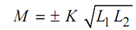## Induced emf Assignment Help

Assignment Help: >> Two Isolated Coils - Induced emf

Induced emf:

Induced emf in coil 1 (because of mutual inductance M between the two coils) suppoaing permeability of mutual flux path is constant

e1 = M (di2 /dt)

also    e1 = N1  (d φ21 /dt)

M  di2 / dt       = N   d φ/ dt

Or  M = N1 (d φ21 / di2)

Assuming the zero initial conditions

M = N1 (φ21 /i2)

Multiply Eq. (1.9) and (1.12)

M2       = ( N12/  i)( N1 21/ i 2)

Now we describe the coefficient of coupling

K = (φ121) = φ21 / φ2

or         K = Useful flux / Total flux

≤ 1

Under ideal conditions, while there is no leakage, then K = 1 (that means. 100% coupling between two coils). In any practical case, K is always less than 1.

From Eq

φ12  = K φ1      and       φ21  = K φ2

Put these values of φ 21 and φ 12 in Eq. (13)

M 2 = (N2 K φ1  /i1  ) (N1  (K φ2 / i1)

= K 2 (N1 φ1 / i1) ( N2 φ2 / i2)

= K2 L1 L2

OrSo M can be positive or negative based upon the winding sense.